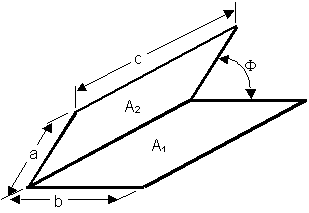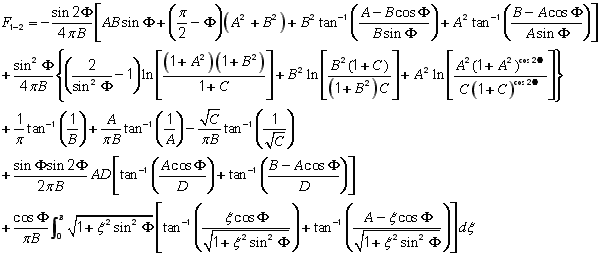# SECTION C Factors From Finite Areas to Finite Areas

## C-16: Two rectangles with one common edge and included angle of Φ.Definitions: A = a/c    B = b/c

C=A2+B2-2ABcosΦ       D=(1+A2sin2Φ)1/2

For Φ = 90°, see C-14

For B approaching infinity, see C-10

Tabular Results:                                Governing Equation:

For Φ= 30°, click here